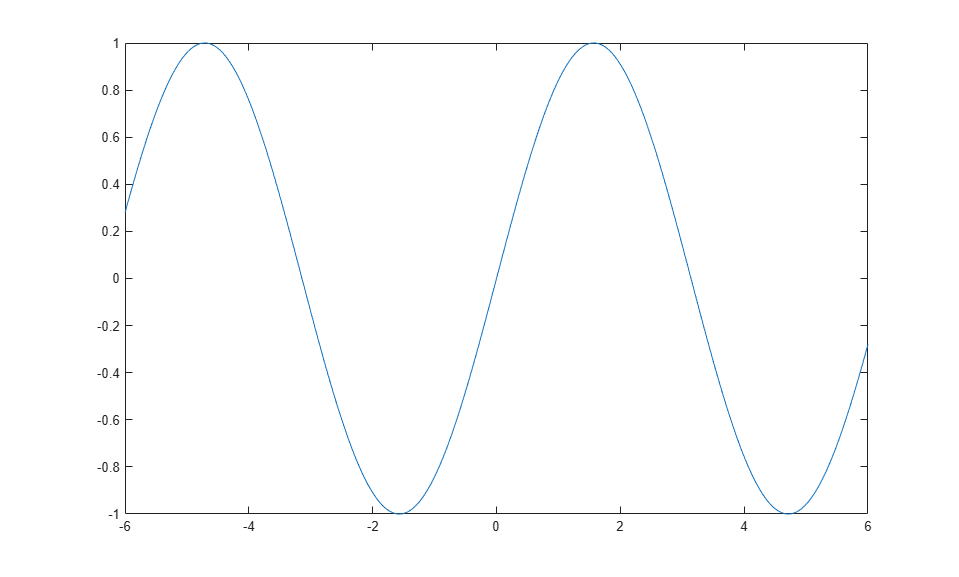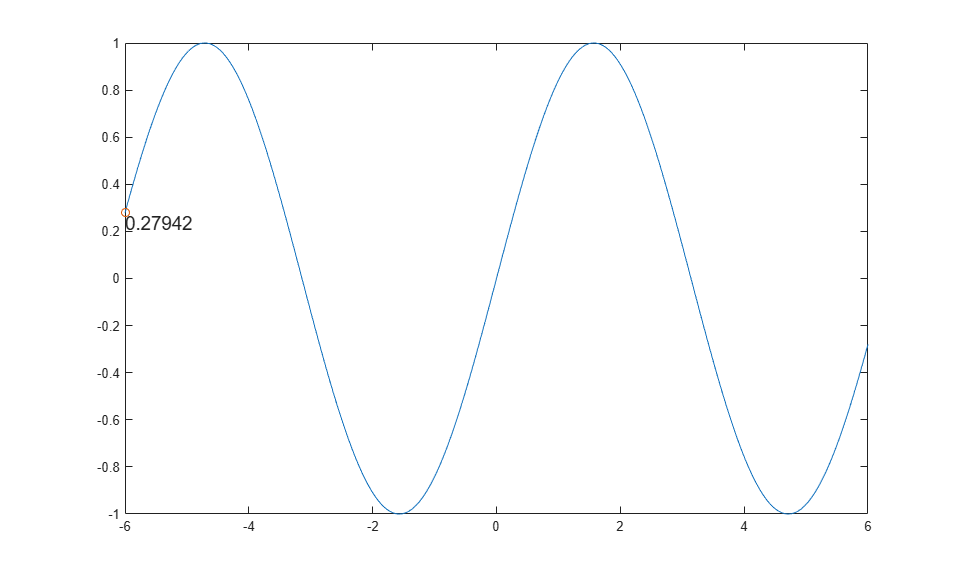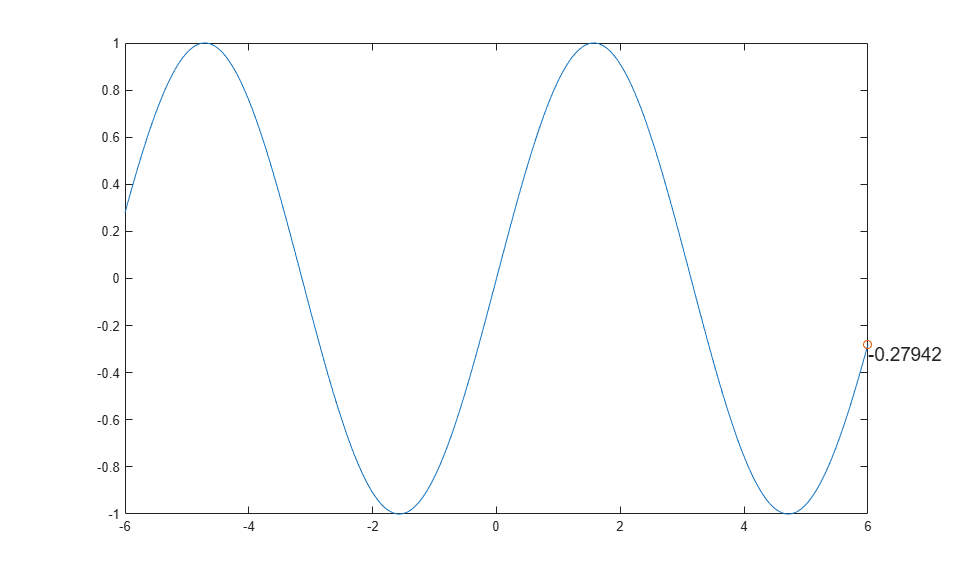# Move Group of Objects Along Line

This example shows how to move a group of objects together along a line using transforms.

Plot a sine wave and set the axis limits mode to manual to avoid recalculating the limits during the animation loop.

```x = linspace(-6,6,1000); y = sin(x); plot(x,y) axis manual ```Create a transform object and set its parent to the current axes. Plot a marker and a text annotation at the beginning of the line. Use the `num2str` function to convert the y-value at that point to text. Group the two objects by setting their parents to the transform object.

```ax = gca; h = hgtransform('Parent',ax); hold on plot(x(1),y(1),'o','Parent',h); hold off t = text(x(1),y(1),num2str(y(1)),'Parent',h,... 'VerticalAlignment','top','FontSize',14); ```Move the marker and text to each subsequent point along the line by updating the `Matrix` property of the transform object. Use the x and y values of the next point in the line and the first point in the line to determine the transform matrix. Update the text to match the y-value as it moves along the line. Use `drawnow` to display the updates to the screen after each iteration.

```for k = 2:length(x) m = makehgtform('translate',x(k)-x(1),y(k)-y(1),0); h.Matrix = m; t.String = num2str(y(k)); drawnow end ```The animation shows the marker and text moving together along the line.

If you have a lot of data, you can use `drawnow limitrate` instead of `drawnow` for a faster animation. However, `drawnow limitrate` might not draw every update on the screen.